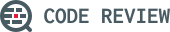0

(click on this box to dismiss)

This is used to see how many closed questions have up, down and neutral voted answers. A single post can be in all three categories if there is an answer which is positive, negative and neutral.Q&A for peer programmer code reviews

``````WITH ClosedPostsVotes as (
SELECT
sum(case when a.Score > 0 then 1 else 0 end) as Up,
sum(case when a.Score < 0 then 1 else 0 end) as Down,
sum(case when a.Score = 0 then 1 else 0 end) as Neutral
FROM
Posts p
inner join
Posts a on a.ParentId = p.Id
WHERE
p.ClosedDate is not null
GROUP BY
p.Id
)

SELECT
count(*) as Questions,
sum(case when v.Up > 0 then 1 else 0 end) as Up,
sum(case when v.Down > 0 then 1 else 0 end) as Down,
sum(case when v.Neutral > 0 then 1 else 0 end) as Neutral
FROMHold tight while we fetch your results